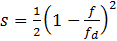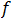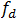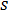# DeviationSquared

Model ElementDeviationSquared

DeviationSquared

## Description

DeviationSquared computes the following value:(1)where:is the value of a ValueAtTime response.is its desired value.is the error in the function.

The functionis computed by another response.

## Attribute Summary

Name Property Modifiable by Command? Designable
label Str ()
measuredValue Response ()   Yes
targetValue Double ()
scale Double (1)

## Usage

DeviationSquared of a particular response from a target value
DeviationSquared (measuredValue=expressionString, targetValue= double, optional_attributes)

## Attributes

measuredValue
A response of type ValueAtTime.
The ValueAtTime response whose deviation you are trying to measure.
measuredValue is mandatory.
targetValue
Double ()
Target value of the response.
targetValue is mandatory.
Optional Attribute
label
String
Specifies the name of the DeviationSquared object.
This attribute is optional. When not specified, MotionSolve creates a label for you.
scale
Double
Specifies the scale factor to scale the DeviationSquared value. If not specified, it defaults to 1.0.
This attribute is optional.

## Example:

# Deviation of the signal DX(22,11,33) at time=2 seconds from a desired value of 4.0

# Compute the ValuaAtTime response
f = ValueAtTime (label="DX@2", atTime=2, delta=0.1)

# Normalized deviation of the signal from 4
s = DeviationSquared (label="Error_in_DX@2", measuredValue=f, targetValue=4)